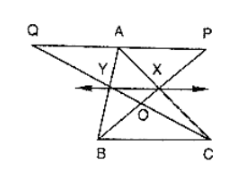Deepak Scored 45->99%ile with Bounce Back Crack Course. You can do it too!

# In figure, X and Y are the mid points of AC and AB respectively, QP ∥ BC and CYQ and BXP are straight lines.

Question:

In figure, X and Y are the mid points of AC and AB respectively, QP ∥ BC and CYQ and BXP are straight lines. Prove that ar(ΔABP) = ar(ΔACQ).Solution:

Since X and Y are the mid points of AC and AB respectively.

Therefore, XY ∥ BC

Clearly, triangles BYC and BXC are on the same base BC and between the same parallels XY and BC

∴ ar(ΔBYC) = ar(ΔBXC)

⇒ ar(ΔBYC) − ar(ΔBOC) = ar(ΔBXC) − ar(ΔBOC)

⇒ ar(ΔBOY) = ar(ΔCOX)

⇒ ar(ΔBOY) + ar(ΔXOY) = ar(ΔCOX) + ar(ΔXOY)

⇒ ar(ΔBXY) = ar(ΔCXY)  .... (2)

We observed that the quadrilaterals XYAP and XYAQ are on the same base XY and between same parallels XY and PQ.